### X.24.8.2 Maxwell distribution

As regards the atomic medium (for simplicity, we take the case of an inert gas which does not produce molecules), the state attribute of the energy which is attributed to an element (by considering equal masses for all the atoms) is the velocity, and since we are talking about energy, only the modulus of this velocity is relevant. Because we are discussing about the modulus of the atoms velocity, in case of a medium which reaches a certain temperature, we know that there is a distribution of this modulus on the total atoms’ set, that is Maxwell distribution.

Comment X.24.8.2.1: If we are taking into account the definition of the abstract object called distribution, which was mentioned in chapter 2 of the present paper, by also identifying according to this definition, which is the support attribute and which is the distributed attribute, as regards the Maxwell distribution, we shall find that the velocity is the support attribute (independent variable) and the distributed attribute (dependent) is the number of atoms (atomic population) which have the velocity rate ranged within the elementary support interval. Therefore, we cannot talk about the distribution of the atoms velocity (because not the velocity is the distributed attribute), but about the distribution of the number of atoms across the support domain of their velocity modulus. If we actually consider that the distribution depending on velocity is a representation of the atoms impulse distribution, we may say that the Maxwell distribution is in fact a precursor of the energy distribution of the atoms from a medium, in this case, the kinetic energy is the distribution’s support attribute. Also, the support attribute (velocity) in case of Maxwell distribution has the positive line of the axis of real numbers as its range of values, which is therefore a continuous set, whereas the distributed attribute (the finite set of the medium’s atoms) has a positive segment of the set of integers (natural numbers) as its values which is therefore a discrete set. However, because the atoms numbers involved into the distribution are very high, they can be written only by using the scientific notation (with decimal mantissa and exponent), the domain of the distributed values seems to be continuous.

The density of this distribution on an elementary support domain with asize and with the internal reference reaching the value(density which means the number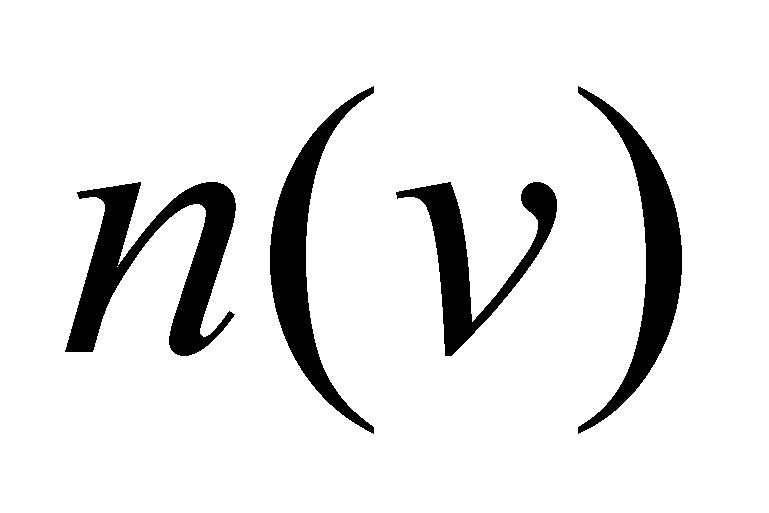of atoms which have its velocity included within the associated support domain) is (according to the textbooks of statistic physics139):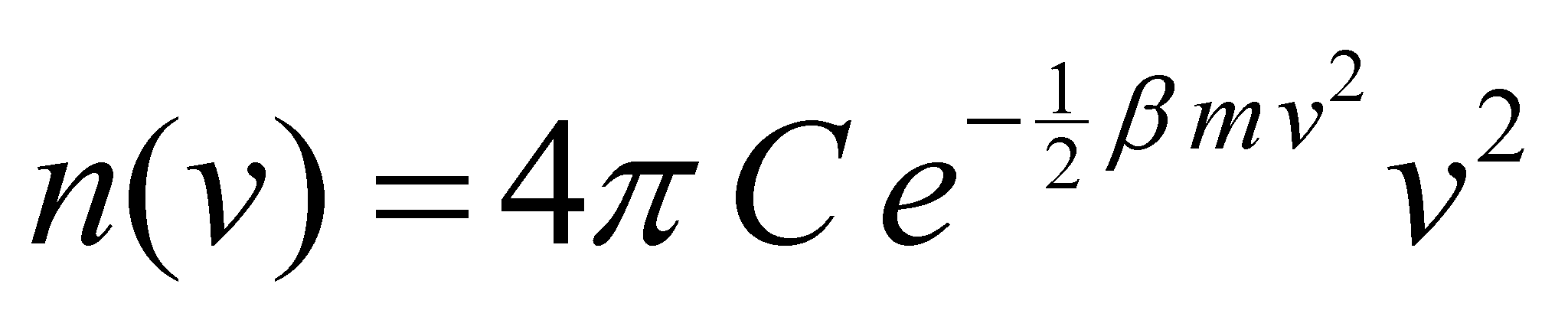(X.24.8.2.1)

where: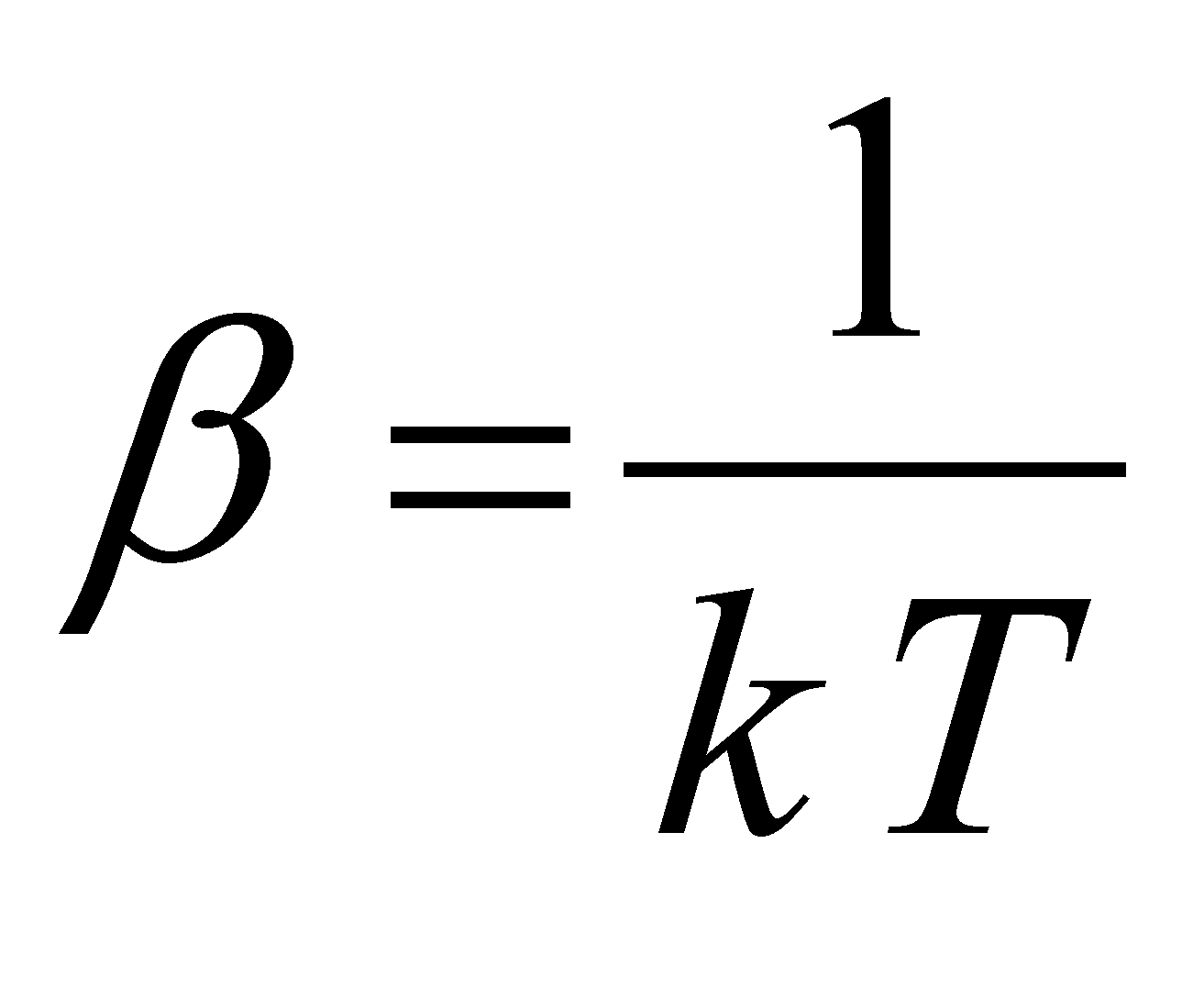(X.24.8.2.2)

and: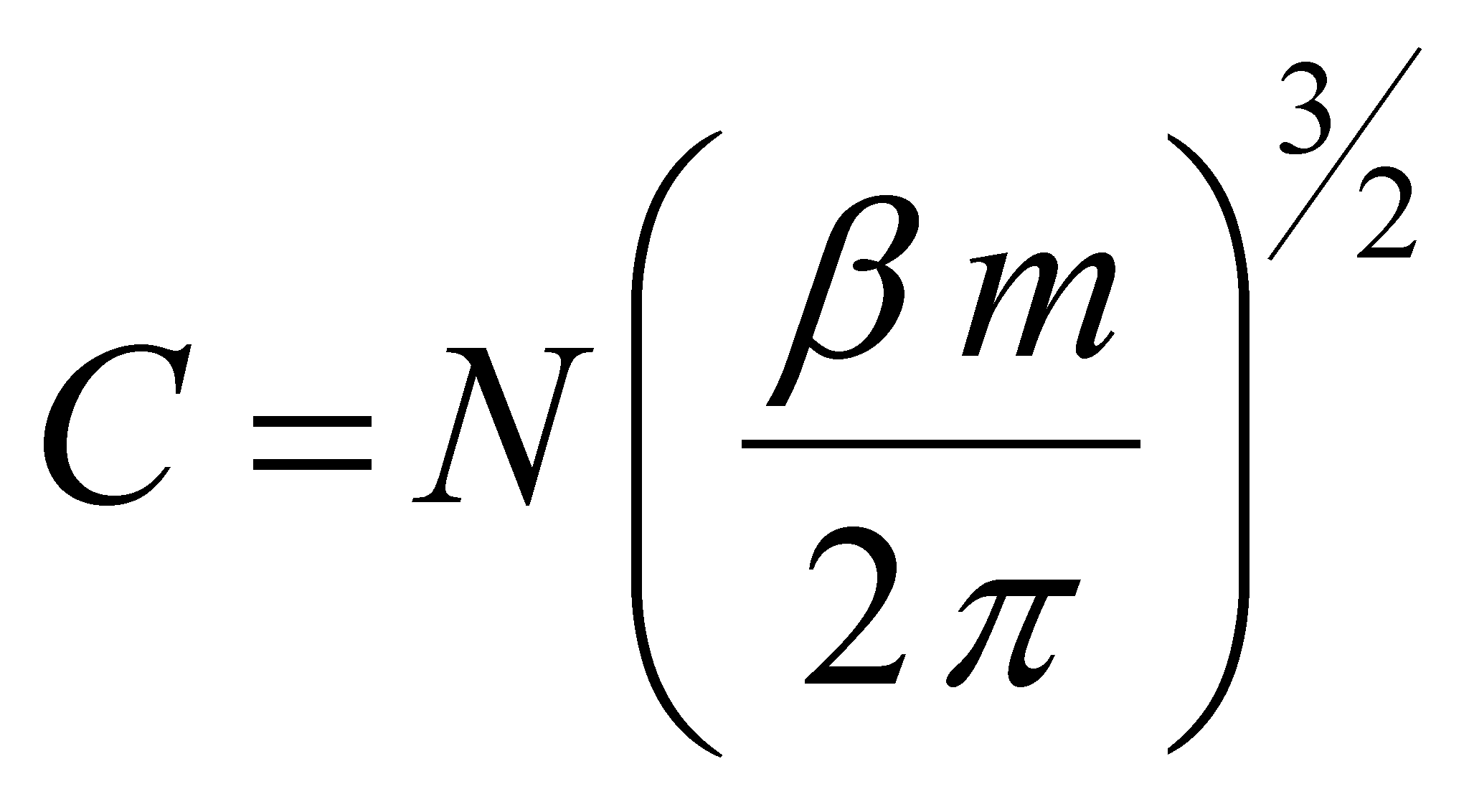(X.24.8.2.3)

N is the total number of atoms inside the medium, m is the atoms mass, T the medium’s temperature and k Boltzmann’s constant. If we are making (similarly to the case of Plank distribution) a variable change in the relation X.24.8.2.1: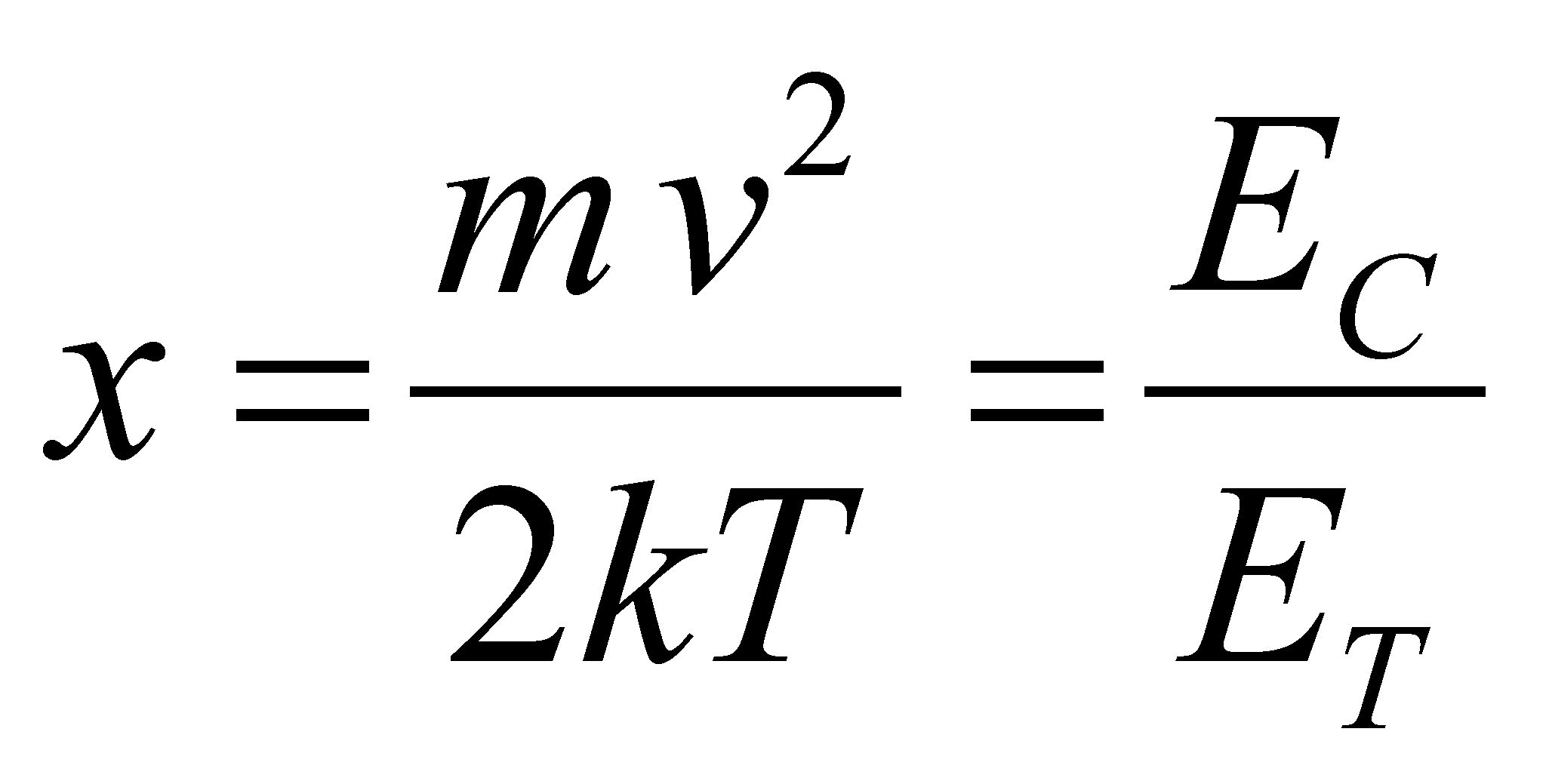(X.24.8.2.4)

where x is also a scalar quantity (ratio of the two energies per element, the kinetic and thermal one), we shall get a normalized Maxwell distribution: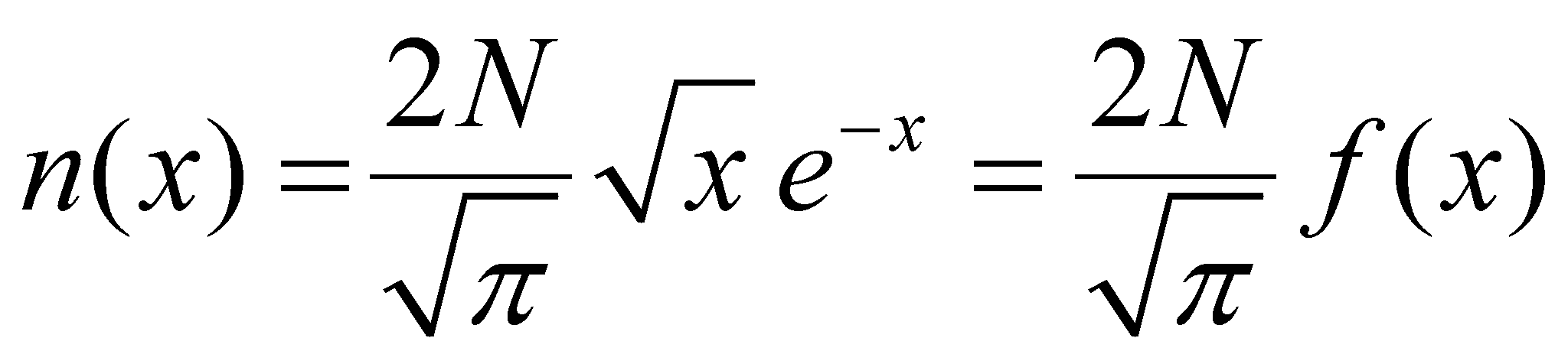(X.24.8.2.5)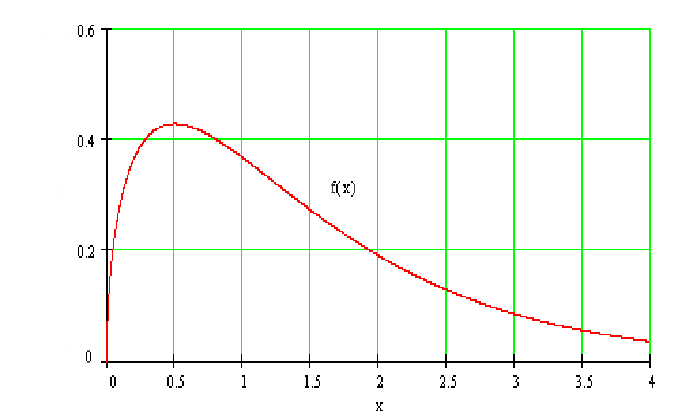Fig. X.24.8.2.1

where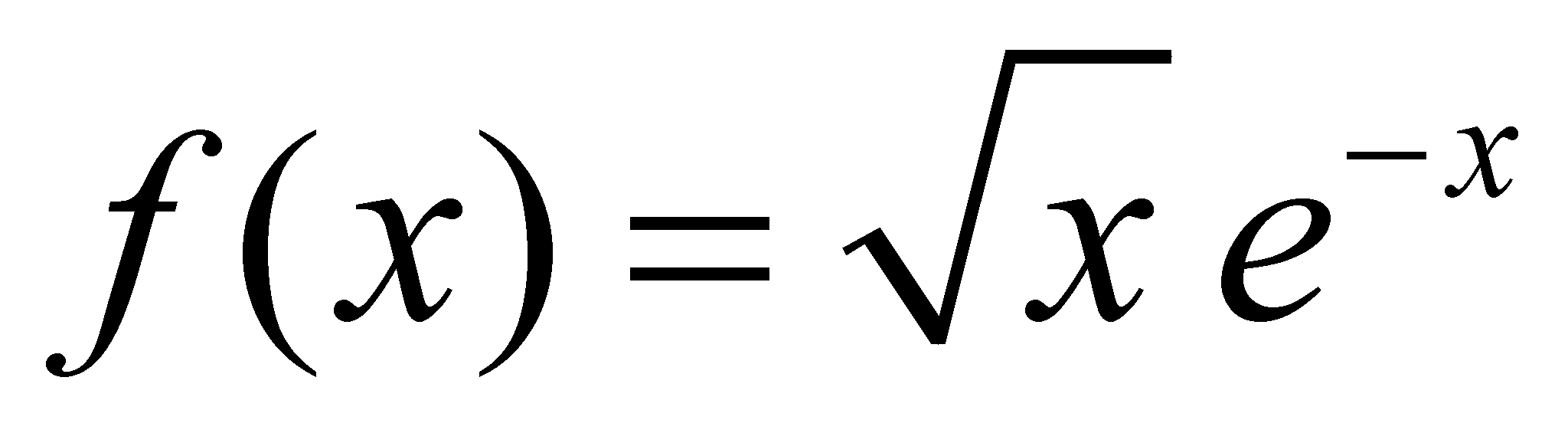is displayed in the figure X.24.8.2.1, with a maximum peak at the value xR=0.5 (the internal reference of the normalized distribution), namely, when the kinetic free translation energy per element of atomic G medium (which corresponds to the distribution’s density of this energy on the atoms set) is half from the energy kT, contained into the photonic medium which is under an equilibrium state. This fact shows us that, in order to exist an equilibrium between the thermal energy density (which has both the component T140 and R), and the density of the kinetic energy deployed by atoms, the rest of the thermal energy (the component R of the photons) must be in equilibrium with the component R of the atoms’ kinetic energy (free energy as well).

Comment X.24.8.2.2: According to the classification of the media in the three fundamental classes S, L and G, now we know that a free translation energy is specific only to the elements of G media, so that the thermal energy from the inner photonic medium shall be distributed to the two possible types of kinetic energy (T and R). In case of the L media, the free translation is not allowed any longer, so that the thermal energy must be in equilibrium only with the kinetic energy (quasi-free) R of the atoms and with the forced vibration energy T. In case of S media, where neither the free motion R is allowed, the thermal energy shall be in equilibrium only with the forced vibration energy (both T and R). The above mentioned assertions are valid for atomic media; for the molecular media, the intramolecular vibrations must be added, each one with specific energy T and R.

As we have seen in the previous sections, the density of the thermal energy (density of the photonic stochastic EF) in a specific medium does not depend on the medium type, but only on its temperature, the thermal energy being exclusively contained into the interstitial medium of the thermal photons. The condition required so that this flux density to be in equilibrium with the density of the kinetic fluxes per element of the atomic or molecular medium, makes however that this equilibrium to be reached in different conditions, at the same amount of thermal energy input, the kinetic parameters of the medium’s elements and the equilibrium temperature depending on the medium type. Otherwise speaking, in a medium (for instance, an atomic one) which is theoretically placed at absolute zero temperature (which means that there are no thermal photons inside it), if we are injecting a quantity of thermal energy (an external thermal photon flux), and in case that the heat losses of the medium are insignificant, this thermal energy shall be distributed due to repeated interactions between the photonic and atomic medium. As a result of this auto-distribution, when the equilibrium state between the photonic stochastic flux and the atomic stochastic flux will be reached, we shall be dealing (depending on the medium type S, L or G) with more energy categories:

• Thermal energy (evidenced by temperature);

• Free T energy (evidenced by pressure and which can be found only into the G media);

• Free R energy (existing only to the G and L media);

• Vibration energy (T and/or R depending on the medium type G, L or S).

Obviously, the sum of all these energy components shall be equal to the thermal energy amount which was initially introduced and which is supposed to be preserved within the medium.

Comment X.24.8.2.3: The above-mentioned discussion on the thermal energy distribution into other energy forms does not depend on the type of atoms which make-up the atomic medium, but a significant remark must be done. Depending on the heat amount which is inserted into the atomic medium, as a result of its distribution into all the possible above-mentioned energy forms, the atom type which creates the medium is very important as regards the medium class (S, L, G) which would result at the moment when the thermal equilibrium is reached. This dependence is due to the uneven spatial distribution (except the atoms of the inert gases) of the electronic orbitals placed on the last layer (the external one), distribution which will produce one or more preferential directions of the future interactions with the other atoms. The more directional and powerful these interactions will be, the more reduced will be the means of subsequent rotation of its elements (for example, the case of the hardly fusible materials or the carbon).

We must clearly underline that the Maxwell distribution is valid only for G media, because, as we have mentioned before, only these media can have a free translation of the medium’s elements. As for the other class of media (S, L), only forced translations (vibrating ones, because there are permanent interactions between elements) can be found. The fact that the distribution of the velocity rates was established as regards the atoms or molecules of some solids or liquids, it does not mean that that particular distribution was valid during the vapour phase of those substances.

Comment X.24.8.2.4: It couldn’t be different anyway, because the velocity selector used for the determination of the atomic or molecular speed rates runs only with atomic or molecular beams (beams of the single elements of the substance) generated by the gaseous phase of the trial substance.

139  F. Reif - Cursul de fizică Berkeley vol. V - Fizică statistică, Editura Didactică şi Pedagogică - Bucureşti, 1983

140 Attention ! Do not mistake the symbol T of the translation (normal font) with the symbol T of the temperature (italics).